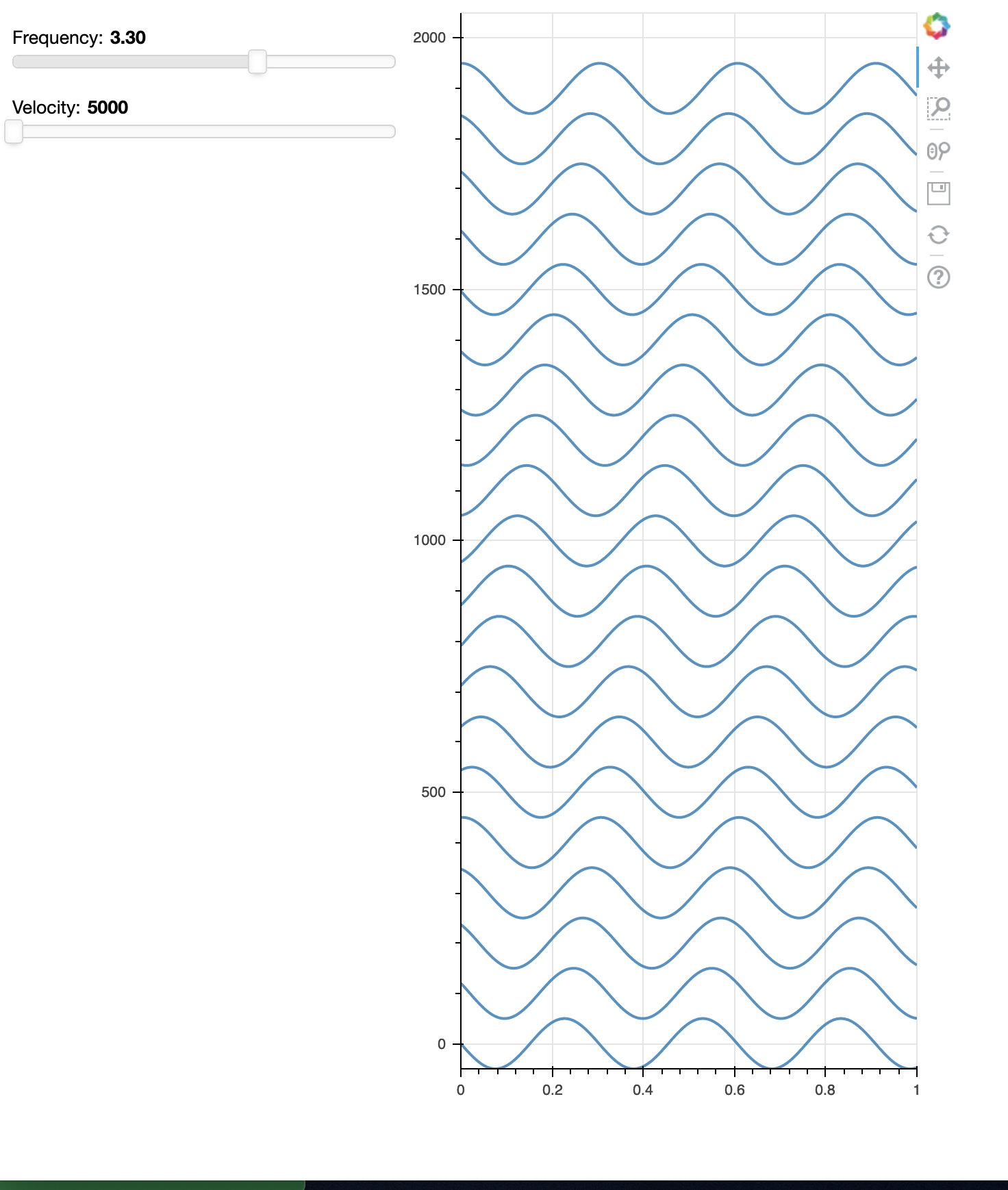# multiline plot with automatic offset (EEG or seismic data style)

Folks, to draw attached, I have to coerce each line with an offset and a scale appropriate to the offset. Is there a way to move the line without rescaling and adding a dc offset?
The `y` list of sine waves has to be scaled by dx and a dc offset of dx*i added. Would be great to just keep `y` as sine waves and then

tell the plotting tool (“multi_line” for example) dx=1 or whatever…

Thanks,

Sridhar

N = 1000

dt = 0.001

Ntrc = 20

dx = 100

v0=5000

f0 = 3

k0 = f0/v0

# time and y(t) for Ntrc sine waves

t = [np.linspace(0, 1, N) for i in np.arange(Ntrc)]

y = [(dx/2)np.sin(2 * np.pi * (k0 * dx * i - f0 * t)) + dxi for i in np.arange(Ntrc)]